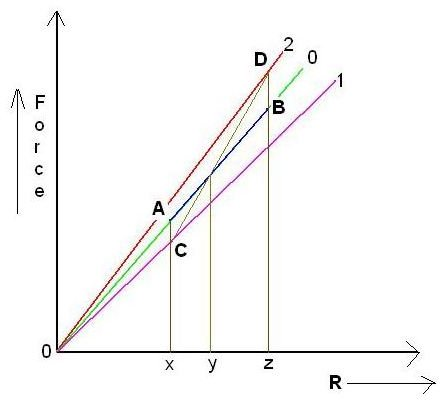# How does a speed governor work – Learn the basics of proportional control

## Introduction

In our previous article we learned about the basics of a governor and the importance of governor mechanism on board ships for main engine as well as auxiliary engines. We will learn how does a speed governor work and also about the simple proportional control used for marine governors though the theory is equally applicable to governors of all types.

## Simple Proportional Control

If you remember from our last discussion, the centrifugal force of the flyweight rotation of the direct action governor was balanced by the spring force. If that arrangement is represented in the form of a mathematical equation it comes to the following

Mώ2R = S

Where M is the mass of the flyweights, omega is the angular speed of rotation of the arrangement measured in radians per second, R is the radius of rotation which is equal to the distance between the governor spindle and the flyweights and S is the controlling force. The left hand side of the equation is also known as the disturbing force, so for equilibrium to exist the controlling force should be equal to disturbing force.

Another thing to be noted from the above equation is that M is constant so it means that the disturbing force is a function of the angular rotation speed as well as the radius R. when the engine is running at a constant speed, meaning thereby that omega is constant, the only variable quantity left is R or radius of rotation.

This situation has been plotted in the form of a graph shown below wherein three different lines are plotted for three different fixed speeds represented by 1, 2 and 3 respectively and the controlling force shown along the vertical axis.It can be imagined that for a given speed let us say that represented by line 0, the value of R should change in proportion to the change in controlling force if the speed has to be along the line 00

Let us take a position where R = y on the horizontal axis. Now if the change in load of the engine causes the value of R to vary in the region of x and z on the same axis, the controlling force should ideally follow the line AB on the 00 line (represented by blue coloured patch on the otherwise green line).

But does it actually happen in such a manner? Well actually not!

In the real world, the controlling force would rather follow the line CD rather than following the line AB and the explanation for this is as follows. If the load increases suddenly the fuel input to the engine would be increased by the governor but the equilibrium speed will not be at A but at C. Similarly the reverse applies for sudden decrease in load and the equilibrium would be attained at D rather than B.

The net effect of all this is that there is a difference between the ideal value and desired value whenever a load change occurs and this difference is known as “offset”. When this offset is relatively large in magnitude the governor is said to be having a coarse behaviour. Similarly if the offset is minimal for a given load change, the governor has a fine control characteristic.

This offset is one of the main disadvantages of the proportional control system, although otherwise it is quite a simple mechanism to implement and understand. In our next article we will start learning about various other governor characteristics such as droop and so forth.